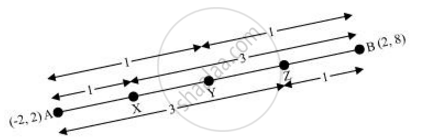# Find the Coordinates of the Points Which Divide the Line Segment Joining A (- 2, 2) and B (2, 8) into Four Equal Parts. - Mathematics

Find the coordinates of the points which divide the line segment joining A (- 2, 2) and B (2, 8) into four equal parts.

#### Solution 1From the figure, it can be observed that points P, Q, R are dividing the line segment in a ratio 1:3, 1:1, 3:1 respectively.

Coordinates of P =

((1xx2+3xx(-2))/(1+3),(1xx8+3xx2)/(1+3))

= (-1, 7/2)

Coordinates of Q = ((2+(-2))/2, (2+8)/2)

= (0,5)

Coordinates of R = ((3xx2+1xx(-2))/(3+1), (3xx8+1xx2)/(3+1))

=(1,13/2)

#### Solution 2

The coordinates of the midpoint (x_m,y_m) between two points (x_1,y_1) and (x_2,y_2) is given by,

(x_m,y_m) = (((x_1 + x_2)/2)"," ((y_1 + y_2)/2))

Here we are supposed to find the points which divide the line joining A(-2,2) and B(2,8) into 4 equal parts.

We shall first find the midpoint M(x, y) of these two points since this point will divide the line into two equal parts.

(x_m, y_m)  = (((-2+2)/2)","((2+ 8)/2))

(x_m, y_m) = (0,5)

So the point M(0,5) splits this line into two equal parts.

Now, we need to find the midpoint of A(-2,2) and M(0,5) separately and the midpoint of B(2,8) and M(0,5). These two points along with M(0,5) split the line joining the original two points into four equal parts.

Let M_1(e,d) be the midpoint of A(−2,2) and M(0,5).

(e,d) = (((-2 + 0)/2)"," ((2 +5)/2))

(e,d) = (-1, 7/2)

Now let M_2(g,h) bet the midpoint of B(2,8) and M(0,5).

(g,h) = ((2 +0)/2)","((8 + 5)/2)

(g,h) = (1, 13/2)

Hence the co-ordinates of the points which divide the line joining the two given points are (-1, 7/2), (0, 5) and (1, 13/2)

Concept: Section Formula
Is there an error in this question or solution?
Chapter 7: Coordinate Geometry - Exercise 7.2 [Page 167]

#### APPEARS IN

NCERT Class 10 Maths
Chapter 7 Coordinate Geometry
Exercise 7.2 | Q 9 | Page 167
RD Sharma Class 10 Maths
Chapter 6 Co-Ordinate Geometry
Exercise 6.3 | Q 40 | Page 30

Share